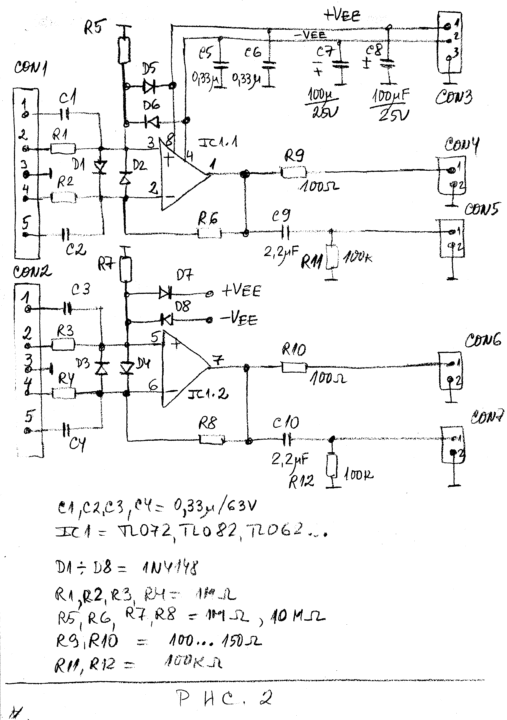# Low Cost High Impedance Buffers for Oscilloscopes and Voltmeters

3450

### Dual buffer with differential inputs

Sometimes we should capture and observe with oscilloscope differential signals. Most of the oscilloscopes need special differential probes or pre-amplifiers in order to capture these signals. Figure 2 presents the schematic diagram of the buffer with differential inputs.

The buffer is with two channels.Figure 2. Schematic diagram of the high impedance buffer with two channels and with unity gain for each channel. The buffer is with differential inputs.

We can use dual operational amplifiers as TL072, TL082, TL062 or similar. The buffer is with input resistance above 1MOhm. The input resistance depends mainly on resistors R1, R2, R3 and R4. If we have R1 = R2 = R3 = R4 = 1MOhm the voltage gain is +1. If we have R1 = R2 = 1MOhm and R3 = R4=10MOhm the voltage gain is +10.

Preferably, the output of the buffers from Figure 1 and Figure 2 should be loaded with resistance higher than 10 kOhm.